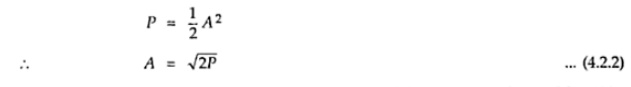Home | | Analog and Digital Communication | Concept of Binary Phase Shift Keying to BPSK, 8 PSK, 16 PSK

# Concept of Binary Phase Shift Keying to BPSK, 8 PSK, 16 PSK

In binary phase shit keying (BPSK), binary symbol ‘1’ and ‘0’ modulate the phase of the carrier.

CONCEPT OF BINARY PHASE SHIFT KEYING TO BPSK, 8 PSK, 16 PSK

CONCEPT OF BINARY PHASE SHIFT KEYING:

Binary Phase Shit Keying(BPSK)

Principle o BPSK

In binary phase shit keying (BPSK), binary symbol ‘1’ and ‘0’ modulate the phase of the carrier. Let the carrier be,‘A’ represents peak value of sinusoidal carrier. In the standard 1Ω load register, the power dissipated will beWhen the symbol is changed, then the phase o the carrier is changed by degree (π radians)

Consider for exampleGraphical Representation of BPSK Signal

Fig 4.2.1 shows binary signal and its equivalent signal b(t).As can be seen from Fig. 4.2.1 (b), the signal b(t) is NRZ bipolar signal. This signal directly modulated carrier cos(2πf0t)The BPSK signal can be generated by applying carrier signal to the balanced modulator.

The baseband signal b(t) is applied as a modulating signal to the balanced modulator. Ig 4.2.2 shows the block diagram of BPSK signal generator.

The NRZ level encoder converts the binary data sequence into bipolar NRZ signal.

Reception of BPSK Signal

Fig 4.2.3 shows the block diagram of the scheme to recover baseband signal from BPSK signal. The transmitted BPSK signal is,Note here that we have neglected the amplitude, because we are only interested in the carrier of the signal.Bandwidth of BPSK SignalThus the minimum bandwidth of BPSK signal is equal to twice of the highest frequency contained in baseband signal.

Study Material, Lecturing Notes, Assignment, Reference, Wiki description explanation, brief detail
Analog and Digital Communication : Concept of Binary Phase Shift Keying to BPSK, 8 PSK, 16 PSK |